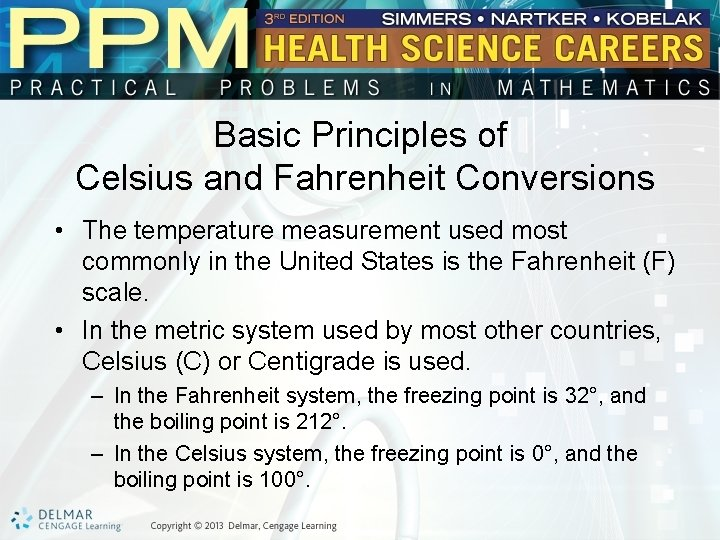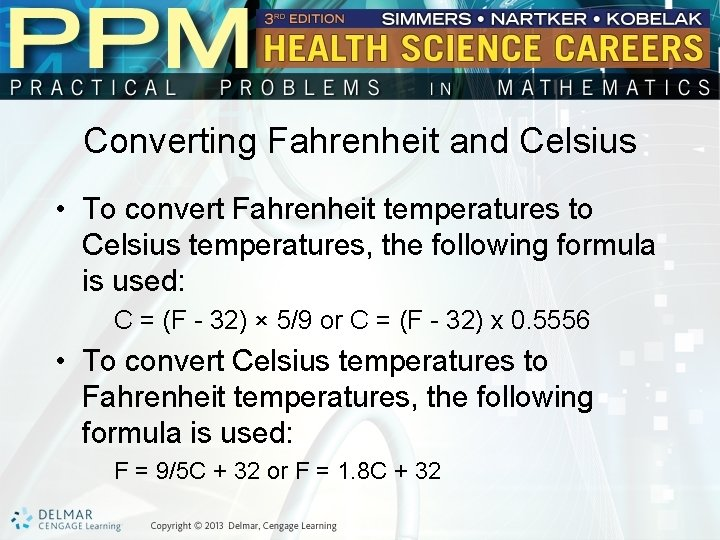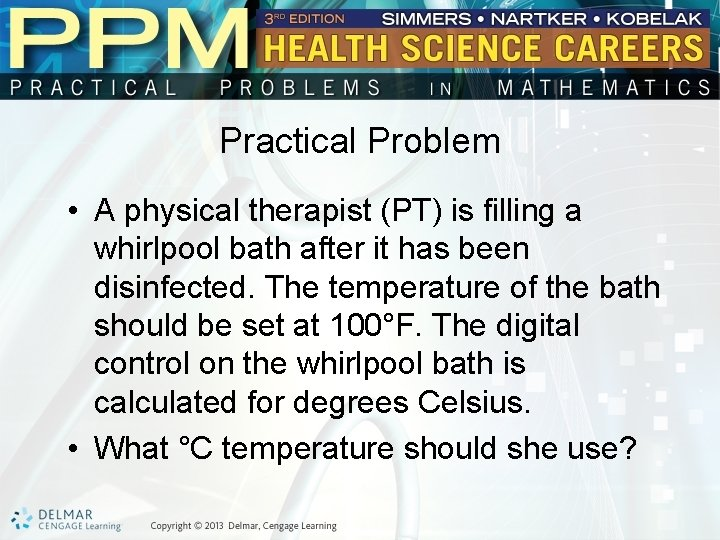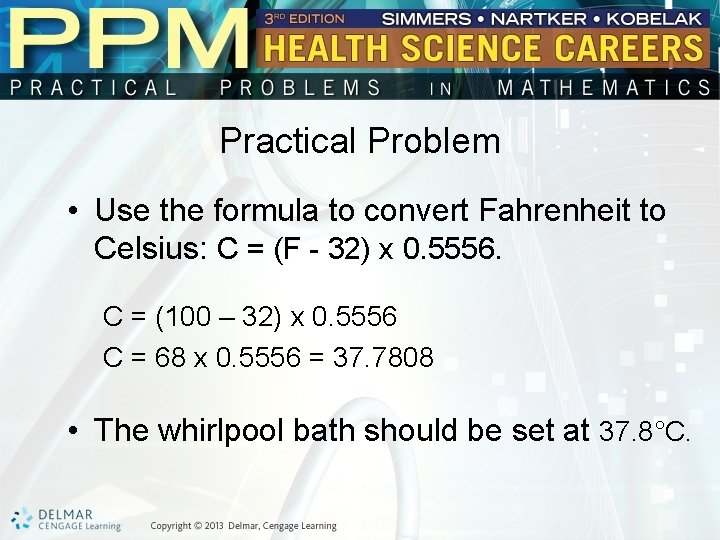# Unit 25 Celsius and Fahrenheit Basic Principles of

• Slides: 6Unit 25 Celsius and FahrenheitBasic Principles of Celsius and Fahrenheit Conversions • The temperature measurement used most commonly in the United States is the Fahrenheit (F) scale. • In the metric system used by most other countries, Celsius (C) or Centigrade is used. – In the Fahrenheit system, the freezing point is 32°, and the boiling point is 212°. – In the Celsius system, the freezing point is 0°, and the boiling point is 100°.Converting Fahrenheit and Celsius • To convert Fahrenheit temperatures to Celsius temperatures, the following formula is used: C = (F - 32) × 5/9 or C = (F - 32) x 0. 5556 • To convert Celsius temperatures to Fahrenheit temperatures, the following formula is used: F = 9/5 C + 32 or F = 1. 8 C + 32Celsius and Fahrenheit • Example: Convert 60°C to degrees Fahrenheit. – Use the formula: F = 1. 8 C + 32 F = (1. 8 x 60) + 32 F = 108 + 32 F = 140° 60°C = 140°FPractical Problem • A physical therapist (PT) is filling a whirlpool bath after it has been disinfected. The temperature of the bath should be set at 100°F. The digital control on the whirlpool bath is calculated for degrees Celsius. • What °C temperature should she use?Practical Problem • Use the formula to convert Fahrenheit to Celsius: C = (F - 32) x 0. 5556. C = (100 – 32) x 0. 5556 C = 68 x 0. 5556 = 37. 7808 • The whirlpool bath should be set at 37. 8°C.